# Algebra - math word problems

1. Triangle perimeterCalculate the triangle perimeter whose sides are in ratio 3: 5: 7 and the longest side is 17.5 cm long.
2. ParkingAt a building parking, 245 spaces are for cars, 56 are for vans and the rest are for buses. If 14% of all parking spaces are for buses, how many parking spaces are there in the building? How many spaces are for buses?
3. Tractors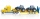5 tractors will plow the field for 8 hours. In 2 hours, 1 tractor broke down. How long do the remaining tractors complete the plow?
4. Three points 2The three points A(3, 8), B(6, 2) and C(10, 2). The point D is such that the line DA is perpendicular to AB and DC is parallel to AB. Calculate the coordinates of D.
5. I thinkI think a number. When I multiply it by five, and after that I subtract 477, I get the same number as if I multiplied it twice. What number do I think?
6. DiscountThe dress was discounted by CZK 115, which was 12% of the original price. What is the cost of the dress after the discount?
7. PupilsThere are 27 pupils in the classroom. They can swim 21 and ski nine pupils. Neither swim nor ski three pupils. How many pupils can swim and ski?
8. Temperature 2Sunday's high temperature was 3 degrees higher than Saturday's. On Monday, the temperature fell 5 degrees, then rose 7 degrees on Tuesday and 4 more on Wednesday. Then it fell 17 degrees to a record low of 31 on Thursday. What was the temperature on Saturd
9. CuSO4 mixtureHow many grams of solid CuSO4 we have to add to 450g of 15% CuSO4 solution to produce a 25% solution?
10. Diagonals of a rhombus 2One diagonal of a rhombus is greater than other by 4 cm . If the area of the rhombus is 96 cm2, find the side of the rhombus.
11. Three students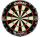Three students independently try to solve the problem. The first student will solve a similar problem with a probability of 0.6, the second student will solve at a probability of 0.55, and the third will solve at a probability 0.04. The problem is resolved
12. Car driver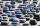The car driver is in town A and is scheduled to be in town B at a specified hour. If it travels at an average speed of 50km/h, it will arrive in city B 30 minutes later. But if he travels at an average speed of 70km/h, he would arrive half an hour earlier
13. The train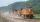The train passed the observer in the 20s and passed through a 1500m tunnel in 2 minutes. Calculate the speed (km/h) and train length.
14. My fatherMy father has a big farm. 6/8 of it were planted with mango trees, 1/2 of the remainder are guava trees and the rest 10 trees are santol trees. What is the number of all trees?
15. Three paintersOne painter would have painted the fence for 15 hours, the second in 12 hours. The plot had to be painted in four hours, so they called the third one, and all worked together. For what time would the third painter paint fence the fence alone?
16. Two pipesHow long will the pool be filled with a double supply pipe if it takes the pool to fill the first pipe by 4 hours longer and the second pipe 9 hours longer than both pipes open at the same time?
17. PeroxideHow many ml 30% of peroxide (H2O2) should be poured into 100ml H2O to give a 20% solution?
18. Dinosaurs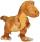More than 30 and less than 60 dinosaurs have met at the pond. A quarter of them bathed and 1/7 saws and the rest gripped. How many were at the pond? How many were there?
19. Children playgroundThe playground has the shape of a trapezoid, the parallel sides have a length of 36 m and 21 m, the remaining two sides are 14 m long and 16 m long. Determine the size of the inner trapezoid angles.
20. Together 3If Sally can paint a house in 4 hours, and John can paint the same house in 6 hours, how long will it take for both of them to paint the house together?

Do you have an interesting mathematical word problem that you can't solve it? Enter it, and we can try to solve it.

To this e-mail address, we will reply solution; solved examples are also published here. Please enter the e-mail correctly and check whether you don't have a full mailbox.

Please do not submit problems from current active competitions such as Mathematical Olympiad, correspondence seminars etc...